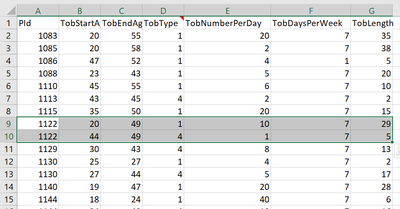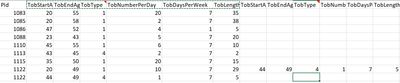## How do I move multiple observations under the same ID to new columns

Hi, I have a large dataset containing some IDs that only have one observation (row) and some have 2-4 observations (rows). Since I'd like to merge this data with another one later, I want to move the extra observations to new columns so that each ID would correspond to a single-row observations. (like the figures below)I tried the codes:

proc transpose data=try out=want (drop=_name_) prefix=TobType_;
by PID;
var TobType;
run;

But these codes can only move one variable at a time and the number of new columns did not match the duplication. I used proc freq to check that the max observations under the same ID are 4, but proc transpose gave me 10 new columns.

I'd like to know if there is a faster way that I can move the entire set of observations to new columns at a time and what is wrong with the codes I used to transpose Tobtype, and why it gives me unmatched numbers of new columns.

1 ACCEPTED SOLUTION

Accepted Solutions

## Re: How do I move multiple observations under the same ID to new columns

Why do you think that you need to make one row before merging with another data set?

I doubt there is anything "wrong" with the transpose, just a misunderstanding of what the procedure does. A single call to proc transpose, depending on the actual data that you have and approach, will turn columns into rows or rows into columns.

Without your actual data I can't say why you get an unexpected number of columns.l

Perhaps you can follow this example. I wouldn't want to do this for a very big set though. This code adds a variable to indicate which row of the 1 to 4 the data is from (I only use 3 rows as I'm somewhat lazy) and then uses that row counter to select which observations to merge with the first one and uses the data set option rename to suffix the variables.

The editor copy / paste and replace text will come in handy to duplicate the 2nd line of the merge and change to use 3 and 4 instead

```data example;
input a b c;
datalines;
1 22 33
1 11 22
1 33 44
2 111 222
2 333 444
;

data need;
set example;
by a ;
retain rowcount;
if first.a then rowcount=1;
else rowcount+1;
run;
data want;
merge need (where=(rowcount=1))
need (where=(rowcount=2) rename=(b=b2 c=c2) )
need (where=(rowcount=3) rename=(b=b3 c=c3) )
;
by a;
drop rowcount;
run;
```

2 REPLIES 2

## Re: How do I move multiple observations under the same ID to new columns

Why do you think that you need to make one row before merging with another data set?

I doubt there is anything "wrong" with the transpose, just a misunderstanding of what the procedure does. A single call to proc transpose, depending on the actual data that you have and approach, will turn columns into rows or rows into columns.

Without your actual data I can't say why you get an unexpected number of columns.l

Perhaps you can follow this example. I wouldn't want to do this for a very big set though. This code adds a variable to indicate which row of the 1 to 4 the data is from (I only use 3 rows as I'm somewhat lazy) and then uses that row counter to select which observations to merge with the first one and uses the data set option rename to suffix the variables.

The editor copy / paste and replace text will come in handy to duplicate the 2nd line of the merge and change to use 3 and 4 instead

```data example;
input a b c;
datalines;
1 22 33
1 11 22
1 33 44
2 111 222
2 333 444
;

data need;
set example;
by a ;
retain rowcount;
if first.a then rowcount=1;
else rowcount+1;
run;
data want;
merge need (where=(rowcount=1))
need (where=(rowcount=2) rename=(b=b2 c=c2) )
need (where=(rowcount=3) rename=(b=b3 c=c3) )
;
by a;
drop rowcount;
run;
```Ksharp
Super User

## Re: How do I move multiple observations under the same ID to new columns

``````/*
The fastest and safest way is using PROC SUMMARY.
*/
proc sort data=sashelp.class out=have;
by age;
run;

proc sql noprint;
select max(n) into :n
from (select count(*) as n from have group by age);
quit;
proc summary data=have;
by age;
output out=want idgroup(out[&n.]  (name sex weight height)=);
run;``````
Discussion stats
• 2 replies
• 327 views
• 0 likes
• 3 in conversation